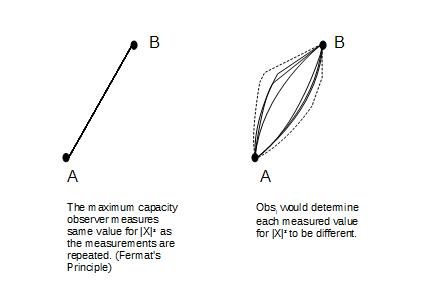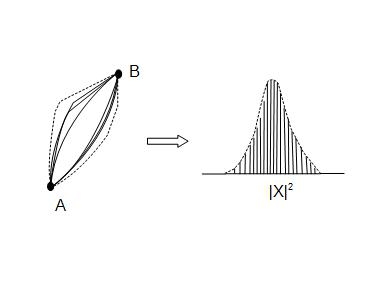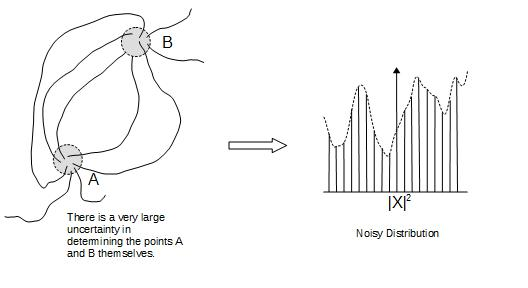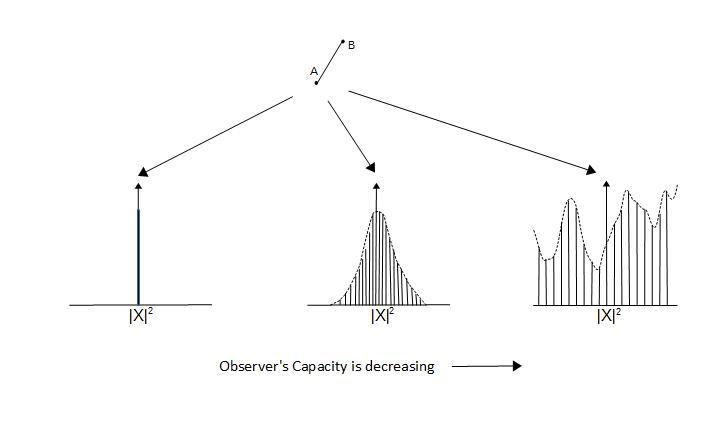# Home

 The  Observers 23rd January 2016 Let us consider the problem of the measurement of the square of the path-length between two nearest points as measured by an observer ObsC situated in Λ∞ plane.  The problem of measurements requires; The measurement of the points "A" and "B" themselves, forming the extremities of the path. The measurement of the path length squared, |X|2, between A and B.  Keep in mind this measurement is important to how the space will be defined.  We can not assume a straight line connecting A and B or in other words a linear path can not be assumed in space between two nearest points.  (This point is beautifully elucidated by the General Theory of Relativity.)     The path-length and the space between A and B,  are not the conventional length and space represented by the Euclidean geometry.  The observer ObsC with maximum capacity, (v/c ≈ 1), measures the path as a straight line.  The quantity |X|2would represent the square of the magnitude of the path between two nearest points in the observer's space.  However an observer of higher capacity (v/c > 1), Obsi, would determine the measured path as non-linear. ( A word of caution, the v/c > 1 here is not referring to tachyons. As we will see later that it means higher spin space. The term v/c > 1 is used only for illustrative purposes.) The situation is described in the following figure:Further as the measurements are repeated for |X|2, the value will not change per ObsC.  However Obsi will determine each measurement made by ObsC to be of different values with all the imperfections in the measurements highlighted.These values for |X|2as determined by Obsi will have a range of values and when plotted together will look like as shown below.The bell shaped curve is assumed, as we know that the processes in nature usually follow the Gaussian distribution1.  We also point out that the path measured by ObsC can not cross the line joining A and B.  In the following picture if the path AB'B was possible then A and B' would be the nearest points instead of A and B.  This would have violated our assumption that A and B were the nearest points to each other per ObsC measurements.So far we have assumed that the points A and B can be determined accurately by ObsC.  If we denote the relative-capacity of an observer by (v/c) where c is the maximum capacity, then to determine A and B with the maximum precision possible in j-space2, the relative-capacity of the observer ObsC has to be v/c ≈ 1.  Please note that we are not in Euclidean space and therefore "v" and "c" should not be confused with conventional velocity notations.     Let us now consider the case for another observer ObsM situated in Λ∞ plane, whose relative- capacity is very low i.e. v/c << 1.  This observer ObsM will not be able to measure the points A and B with the same precision as ObsC.  Consequently even though ObsM may think that the values measured for |X|2 are linear in shape and have identical values, Obsi and ObsC  both shall determine them to be non-linear and varying from each other with a large variation.  The resulting distribution will be very noisy instead of a delta function.  A representative scenario is shown in the diagram below.Our problem was to connect two nearest points.  Different observers provided different measurements each certain that their measurements were complete for the points A, B, and the quantity \X\2.  These measurements were evaluated by Obsi.  Thus the same problem had different results based on measurements made by observers of different capacities.The area under the each curve is unity.  We can also think of a scenario where there is only one observer and three different paths are being measured.  Path-A requires only a single measurement and essentially it is a zero-entropy process (not achievable).  Path-B is a finite entropy process, hence points A and B can be measured precisely (with observer's maximum capacity) but |X|2 varies.  And Path-C is an infinite entropy process where neither points A and B nor the connections between them can be measured precisely (observer in an inertial frame, or classical Newtonian mechanics).     The path AB can be represented by the closed interval [0,1] for ObsC or ObsM.  However per Obsi criterion the interval is open and represented by ]0,1[.          To summarize, we have defined the problem of measurement.  The definition is independent of the observer's measurement-metric.  We have discussed the existence of the curvature in j-space which must be followed by (t, x, y, z, m, q) or the space-time metric corresponding to the macroscopic observer in Λ∞ plane.  We have also discussed the statistical nature of the measurements made by the observers of various capacities. We note that the actual shortest path between two points was never determined and it was not part of the measurements made by the either observer.  These are important issues needed to be resolved before discussing the EPR paradox and the "Copenhagen Interpretation" of Quantum Mechanics. Why are we measuring the quantity |X|2 ?  Why not measure the path-length |X| itself ? _________________ 1. The Gaussian or Normal distribution along with Cauchy and Lèvy distributions, is known as a stable distribution. The stable distributions follow the linearity property. For example, two Gaussian distributions when derived independently for the same random variable and then combined, will provide another Gaussian distribution. These distributions are not analytically expressible i.e. the relationship between the points can not be expressed in form of an equation.  In j-space such distributions represent PE1j measurements or stable structures, in Λ∞ plane. There are distributions such as Chi-Square (bounded at zero), who approach Gaussian distribution under asymptotic limits and hence stability.     We realize that we are associating linearity and hence "addition" to the "stability of the structure" in j-space. This is the first time we have introduced an arithmetic operation in our measurement space and it certainly did not come from the analytical expressions. We can also introduce "subtraction" based on linearity for the low-information or high quantum number states. However we still do not know about operations such "multiplication" and "division". The VT-Symmetry is a requirement for the arithmetic operations in j-space. 2. The measurement of ObsC  (v/c ≈ 1), are described by Pauli's spin matrices. We need Hamilton's anharmonic coordinates to understand their effect (i.e. finiteness), on the physical measurements made by the macroscopic observer ObsM in Λ∞-plane. Previous Blogs:      "What happens and what is observed" - Bertrand Russell, ABC of Relativity. "When a blind beetle crawls over the surface of a curved branch, it doesn’t notice that the track it has covered is indeed curved. I was lucky enough to notice what the beetle didn’t notice." - Albert Einstein from "The Ultimate Quotable Einstein" by Alice Calaprice. "If one were to think of the physical world as a stage, then the actors would be not only electrons, which are outside the nucleus in atoms, but also quarks and gluons and so forth-dozens of kinds of particles-inside the nucleus. And though these "actors" appear quite different from one another, they all act in a certain style-a strange and peculiar style-the "quantum" style." - Richard Phillips Feynman,  QED: The Strange Theory of Light and Matter.Best viewed with Internet Explorer